If you want to plot trend lines in the RadHtmlChart for ASP.NET AJAX control and get a basic understanding on statistics terms like linear/logarithmic regression lines, ordinary least square method and r-squared measurement, do not miss the opportunity to examine this blog post to the end.

## What is a Linear Regression?

The term “linear regression” (Figure 1) refers to an approach in statistics, related to modelling the relationship between a dependent variable/variables (usually denoted by Y) and an independent variable (usually denoted by X).

When we have an equation with a single X variable ,we have a simple linear regression. If we have multiple X variables, we have a multivariate linear regression.

Regression lines are also known as trend lines, and there are different types such as linear, logarithmic, exponential and so on.  Because the linear regression with a single independent variable is the basic case of the regression modelling, this is the one we will examine.

Figure 1: A sample linear regression plot in MS Excel that shows the relationship between the quantity and yield of bonds.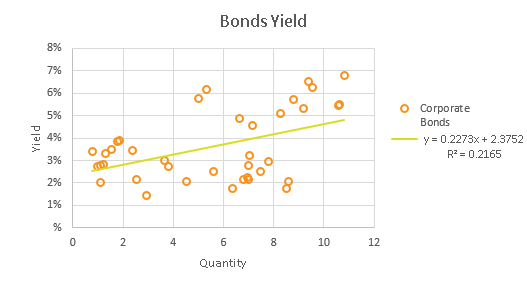You can compare the chart from MS Excel above (Figure 1) with our final result from Telerik ASP.NET Chart at the end of the blog post (Figure 3).

## Use Cases of the Linear Regression

Linear regressions can be used to forecast/predict values by developing a model over a data set of observation. Say you have the results from a student survey about the number of coffee cups drunk before attending an exam and the marks after the exam. Now you can find a potential relationship between both variables, so you can predict the exam mark of a student that will drunk "n" cups of coffee.

In the case of a multivariate regression, you can also determine which independent variables have or have no effect over the dependent one. For example, you can develop a bank score card that illustrates which characteristics (for example, age, salary, marital status and so on), and how they are related to the solvency of a potential creditor.

Before proceeding further, we will also cover another statistics term, tightly related to the linear regression subject–the ordinary least squares method.

### The Ordinary Least Squares Method (OLS)

The OLS method purpose is to estimate such parameters (α - the slope and β - the intercept) of the linear regression model (y = α*x + β) that the sum of squared residuals (i.e., the sum of the differences between the observed and predicted responses in a data set) is the minimum.

Imagine we have a bunch of random points, and we plot a straight line through it. Now our target is to find the minimum aggregate of the vertical distances between the points and the line which is illustrated by the estimates of the α and β parameters as follows: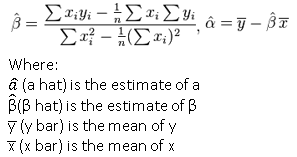To get a better understanding of the above formulae we will create a sample data set of (X,Y) points in MS Excel and calculate the corresponding parameters as illustrated in Table 1.

Table 1: Ordinary least squares method formulas in MS Excel with a sample data set.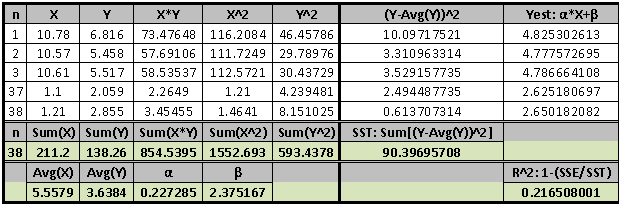For the moment ,we will pay attention to the steps that create only the left side of the table:

1. Create these columns - X*Y; X^2; Y^2
2. Calculate these values: Sum(X); Sum(Y); Sum(X*Y); Sum(X^2); Sum(Y^2); Avg(X); Avg(Y)
3. Calculate α = [n*Sum(X*Y) - Sum(X)*Sum(Y)]/[n*Sum(X^2)-Sum(X)^2]
4. Calculate β = Avg(Y) - α*Avg(X)

Since we have the necessary formula sand logic in MS Excel, we can simply transpose that in .NET.

### OLS in .NET

We are going to use a DataTable data source type for our current purpose, but the same logic can be applied to any other data source, as well:

1. We will start with the creation of the auxiliary columns from step 1 through the Add method of the DataTable instance
2. Then ,we continue with the calculation of the SUM/AVG functions through the Compute method
3. Last, we substitute these values in the formulae from step 3 and 4 above

Let’s take a look at the C# code below to make things clearer:

Example 1: A part of the OrdinaryLeastSquares method that shows how to calculate slope and intercept.

`dt.Columns.Add(``"__XY"``, ``typeof``(``double``), ``string``.Format(``"{0} * {1}"``, xField, yField));`
`dt.Columns.Add(``"__X2"``, ``typeof``(``double``), ``string``.Format(``"{0} * {0}"``, xField));`
`dt.Columns.Add(``"__Y2"``, ``typeof``(``double``), ``string``.Format(``"{0} * {0}"``, yField));`

`double` `xSum = (``double``)dt.Compute(``string``.Format(``"SUM([{0}])"``, xField), ``""``);`
`double` `ySum = (``double``)dt.Compute(``string``.Format(``"SUM([{0}])"``, yField), ``""``);`

`double` `xAvg = (``double``)dt.Compute(``string``.Format(``"AVG([{0}])"``, xField), ``""``);`
`double` `yAvg = (``double``)dt.Compute(``string``.Format(``"AVG([{0}])"``, yField), ``""``);`

`double` `xySum = (``double``)dt.Compute(``"SUM([__XY])"``, ``""``);`
`double` `x2Sum = (``double``)dt.Compute(``"SUM([__X2])"``, ``""``);`
`double` `y2Sum = (``double``)dt.Compute(``"SUM([__Y2])"``, ``""``);`

`int` `n = dt.Rows.Count;`

`double` `slope = (n * xySum - xSum * ySum) / (n * x2Sum - xSum * xSum);`
`double` `intercept = yAvg - slope * xAvg;`

Ok, we have created the regression model and can proceed further by determining how good it is, thanks to the r-squared measure.

### R-Squared

R-squared, a.k.a. the coefficient of determination is a statistical measure that illustrates how well the data fits to the regression model. The coefficient ranges from 0 to 1, where values close to 1 indicate a good model (the variability of X explains well the variability of Y) while values close to 0 indicate the contrary.

You can see how the coefficient is calculated in Figure 2.

Figure 2: Formulas of the r-squared measure.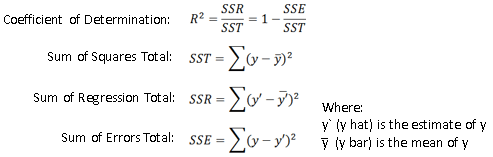Now, we can get back to Table 1 but have a look this time at the right part of it, responsible for the r-squared calculation.

1. Create these columns: (Y-Avg(Y))^2; Yest: α*X+β; (Y-Yest)^2
2. Calculate these values: SST: Sum[(Y-Avg(Y))^2]; SSE: Sum[(Y-Yest)^2]
3. Calculate: R^2 = 1-(SSE/SST)

And the C# code analogue:

Example 2: A part of the OrdinaryLeastSquares method that shows how to calculate the coefficient of determination.

`dt.Columns.Add(``"__SSTField"``, ``typeof``(``double``), ``string``.Format(``"({0} - {1}) * ({0} - {1})"``, yField, yAvg));`
`dt.Columns.Add(``"__Yest_Orig"``, ``typeof``(``double``), ``string``.Format(``"{0} * {1} + {2}"``, slope, xField, intercept));`
`dt.Columns.Add(``"__SSEField"``, ``typeof``(``double``), ``string``.Format(``"({0} - __Yest_Orig) * ({0} - __Yest_Orig)"``, yField));`

`double` `SST = (``double``)dt.Compute(``"SUM([__SSTField])"``, ``""``);`
`double` `SSE = (``double``)dt.Compute(``"SUM([__SSEField])"``, ``""``);`

`double` `rSquarred = 1 - SSE / SST;`

## Plot a Linear Regression with RadHtmlChart

To plot a liner regression in RadHtmlChart with the current example, follow these steps:

2. Place the RegressionModels.cs file in the App_Code folder of your web app/site
3. Include the RegressionModels namespace in the code behind logic of your page
4. Call the CreateRegressionModel.Plot method and pass the required parameters:
2. DataSource: The DataTable data source
3. DataFieldX: The name of the column in the data source that stores the x-values
4. DataFieldY: The name of the column in the data source that stores the y-values
5. RegressionModelType: The type of the regression model

If you wonder what the RegressionModelType parameter is for, here come the custom modifications you can do in this example. You can easily add support for a logarithmic regression by adding a field that calculates the Ln of X and use it instead of the original X field:

`if` `(regressionType == RegressionType.Logarithmic)`
`{`
`    ``dt.Columns.Add(``"__LnX"``, ``typeof``(System.Double));`
`    ``dt.Rows.Cast<DataRow>().ToList().ForEach(r => r.SetField(``"__LnX"``, Math.Log((``double``)r[xField])));`
`    ``xField = ``"__LnX"``;`
`}`

The code above resides inside t
he OrdinaryLeastSquares method.

You can also show the regression model to the legend. Just create the corresponding format string:

`private` `static` `string` `FormatStringEquation(RegressionType regressionType, ``double` `slope, ``double` `intercept, ``double` `rSquared)`
`{`
`    ``string` `XName = ``"X"``;`
`    ``if` `(regressionType == RegressionType.Logarithmic)`
`    ``{`
`        ``XName = ``"Ln(X)"``;`
`    ``}`

`    ``return` `string``.Format(``"Y = {0} * {3} + {1}\\nR-Squared: {2}"``, Math.Round(slope, 4), Math.Round(intercept, 4), Math.Round(rSquared, 4), XName);`
`}`

Then pass it to the Name property of the series:

`        ``string` `equationSeriesName = FormatStringEquation(RegressionModelType, slope, intercept, rSquared);`

`        ``AddRegressionSeries(HtmlChart, estXFieldName, estYFieldName, equationSeriesName);`

`    ``private` `static` `void` `AddRegressionSeries(RadHtmlChart chart, ``string` `xField, ``string` `yField, ``string` `seriesName)`
`    ``{`
`        ``ScatterLineSeries scatterLineSeries1 = ``new` `ScatterLineSeries();`
`        ``scatterLineSeries1.Name = seriesName;`
`...`
`        ``chart.PlotArea.Series.Add(scatterLineSeries1);`
`    ``}`

I think it is high time we saw our final result in Figure 3:

Figure 3: RadHtmlChart that has its second series fits the data of the first series and displays the regression model in the legend.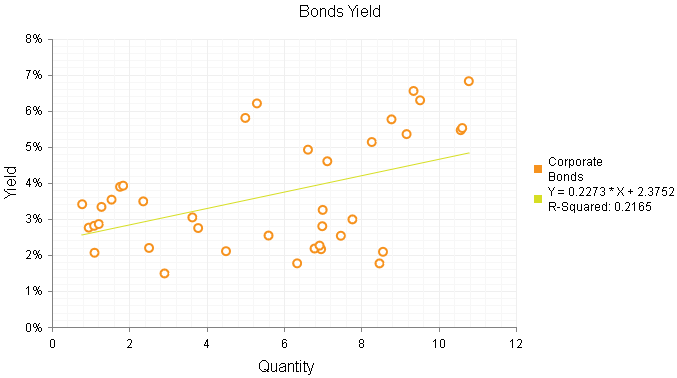## Found the Example Useful?

We went through the basics of a popular approach in statistics–the simple linear regression--and illustrated how to integrate it in the Telerik chart for ASP.NET AJAX control. Please feel free to share your thoughts and feedback. The source code of the demo application is also available in the Plot Regression Models with RadHtmlChart code library.